### Topological linear spaces and related structures (46Axx)

• Article
• ##### Democracy of quasi-greedy bases in $\boldsymbol p$-Banach spaces with applications to the efficiency of the Thresholding Greedy Algorithm in the Hardy spaces $\boldsymbol {H_{p}({\mathbb {D}}^{d})}$• Proceedings of the Royal Society of Edinburgh Section A: Mathematics, First View
• Article
• ##### Interpolation between noncommutative martingale Hardy and BMO spaces: the case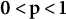$\textbf {0<p<1}$
• Canadian Journal of Mathematics, Volume 74, Issue 6

### Linear function spaces and their duals (46Exx)

• Article
• ##### A weighted Trudinger–Moser inequalities and applications to some weighted $(N,q)-$Laplacian equation in $\mathbb {R}^N$with new exponential growth conditions
• Proceedings of the Royal Society of Edinburgh Section A: Mathematics, First View

### Measures, integration, derivative, holomorphy (all involving infinite-dimensional spaces)

• Article
• ##### A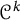$\mathcal {C}^k$ -seeley-extension-theorem for Bastiani’s differential calculus
• Canadian Journal of Mathematics, Volume 75, Issue 1

### Commutative Banach algebras and commutative topological algebras (46Jxx)

• Article
• ##### ON THE ALGEBRAS$\boldsymbol {VN(H)}$ AND$\boldsymbol {VN(H)^{*}}$ OF AN ULTRASPHERICAL HYPERGROUP$\boldsymbol {H}$
• Journal of the Australian Mathematical Society, First View

• Article
• #####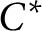$C^*$ -algebra structure on certain Banach algebra products
• Canadian Mathematical Bulletin, Volume 64, Issue 3

### Selfadjoint operator algebras ($C^*$-algebras, von Neumann ($W^*$-) algebras, etc.) (46Lxx)

• Article
• ##### Radius of comparison and mean topological dimension:$\mathbb Z^d$-actions
• Canadian Journal of Mathematics, First View

### Methods of category theory in functional analysis (46Mxx)

• Article
• ##### The generator rank of subhomogeneous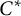$C^*\!$-algebras
• Canadian Journal of Mathematics, Volume 75, Issue 4

### Miscellaneous applications of functional analysis (46Nxx)

• Article
• ##### A note on Mosco convergence in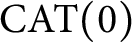$\operatorname {\mathrm {CAT}}(0)$ spaces
• Canadian Mathematical Bulletin, Volume 65, Issue 4